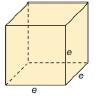Chapter 9.1, Problem 31E### Elementary Geometry for College St...

6th Edition
Daniel C. Alexander + 1 other
ISBN: 9781285195698

#### Solutions

Chapter
Section### Elementary Geometry for College St...

6th Edition
Daniel C. Alexander + 1 other
ISBN: 9781285195698
Textbook Problem
1 views

# A cube is a right square prism in which all edges have the same length. For the cube with edge e , a ) Show that the total area is T = 6 e 2 . b ) find the total area if e = 4 cm. c ) Show that the volume is V = e 3 . d ) find the volume if e = 4 cm.To determine

a)

To prove:

The total area is T=6e2

Explanation

The total area of any solid is the area of all the number of lateral sides that it have, including the area of the top and the bottom of solid.

Thus, the total area (T) of any solid prism with its lateral area (L) and base area (B) is given by the formula.

T=L+2B

Here, we have 2B since, any solid prison will have two bases (One upper part and another the lower part).

Calculation:

Given,

The edge of the right square prism (cube) =e.

The total area of a regular prism with lateral area ‘L’ and base area B is given by the formula

T=L+2B.

First let’s calculate the lateral area of the prism.

The lateral area of the prism with its base perimeter ‘P’ and altitude ‘h’ is given by

L=Ph

Here, the base of the prism is with the square base and of each edge of length ‘e

To determine

b)

To find:

The total area if e=4 cm.

To determine

c)

To prove:

The volume is V=e3.

To determine

d)

To find:

The volume if e=4 cm.

### Still sussing out bartleby?

Check out a sample textbook solution.

See a sample solution

#### The Solution to Your Study Problems

Bartleby provides explanations to thousands of textbook problems written by our experts, many with advanced degrees!

Get Started

#### Find more solutions based on key concepts